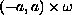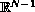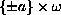Electron. J. Diff. Eqns., Vol. 2003(2003), No. 108, pp. 1-20.

### Symmetry and monotonicity of solutions to some variational problems in cylinders and annuli Friedemann Brock

Abstract:
We prove symmetry and monotonicity properties for local minimizers and stationary solutions of some variational problems related to semilinear elliptic equations in a cylinder, whereis a bounded smooth domain in. The admissible functions satisfy periodic boundary conditions on, and some other conditions. We show also symmetry properties for related problems in annular domains. Our proofs are based on rearrangement arguments and on the Moving Plane Method.

Submitted March 9, 2003. Published October 24, 2003.
Math Subject Classifications: 35J25, 35B10, 35B35, 35B50, 35J20.
Key Words: Variational problem, periodic boundary conditions, Neumann problem, symmetry of solutions, elliptic equation, cylinder, annulus.

Show me the PDF file (301K), TEX file, and other files for this article.

Friedemann Brock